2.2. Fluorescence

Sometimes we have the situation in which the atom or ion is in one of its first m lower-lying levels and then it is photoexcited to some higher-lying level(e.g., by an UV radiation field). Next it decays - either spontaneously or by stimulated emission - back to some different level among the first m levels. We call this process fluorescence.

In this case it is easy to eliminate the levelfrom the linear system of equations (2), reducing its order by one.

Initially, for notation purposes let us define: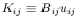(9)

The statistical equilibrium equation (2) for some level l belonging to the first m lower-lying levels is: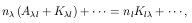(10)

where we have written only the terms involving the level. Whereas the equation for levelwill be: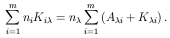(11)

Solving for nin eq. (11), substituting in eq. (10) and introducing the indirect excitation rate from level i to level j through levelas: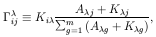(12)

withij= 0; we can now rewrite eq. (10) as,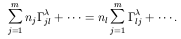(13)

And we have eliminated the equation involving n. Extending this reasoning to eliminate a whole set of µ higher-lying levels is straightforward. One simply replaces the indirect excitation rates in eq. (13) by the corresponding total indirect excitation rates: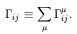(14)